# 3D Shapes Faces Edges Vertices Worksheets

Please check Solutions of 3D Shapes Faces Edges Vertices Worksheets at the end of the questions.1. A cuboid has ________ faces.
a) 8
b) 4
c) 5
d) 6

2. A cuboid has ________ Vertices.
a) 4
b) 5
c) 6
d) 8

3. A cuboid has ________ edges.
a) 6
b) 7
c) 12
d) 8

4. A cube has ________ faces.
a) 4
b) 5
c) 6
d) 8

5. A cube has ________ vertices.
a) 4
b) 5
c) 6
d) 8

6. A cube has ________ edges.
a) 6
b) 5
c) 12
d) 8

7. A cylinder has ________ faces.
a) 4
b) 3
c) 6
d) 5

8. A cylinder has ________ vertices.
a) 0
b) 1
c) 3
d) 2

9. A cylinder has ________ edges.
a) 4
b) 2
c) 3
d) 5

10. A sphere has ________ faces.
a) 1
b) 2
c) 3
d) 4

11. A sphere has ________ vertices.
a) 1
b) 0
c) 2
d) 3

12. A sphere has ________ edges.
a) 0​
b) 2
c) 3
d) 4

13. A cone has ________ faces.
a) 1
b) 2
c) 3
d) 4

14. A cone has ________ vertices.
a) 1
b) 2
c) 3
d) 4

15. A cone has ________ edges.
a) 1​
b) 2​
c) 3
d) 4

16. A triangular prism has ________ rectangular lateral faces and _ triangular bases
a) 3,4
b) 3,2
c) 2,3
d) 4,3

17. A triangular prism has ________ vertices.
a) 2
b) 5
c) 8
d) 6

18. A triangular prism has ________ edges.
a) 7
b) 9
c) 8
d) 6

19. A square pyramid has ________ lateral triangular faces and ________ square base.
a) 3,1
b) 1,3
c) 4,1
d) 1,4

20. A square pyramid has ________ vertices.
a) 4
b) 5
c) 3
d) 6

21. A square pyramid has ________ edges.
a) 7
b) 6
c) 8
d) 5

22. A triangular pyramid has ________ triangular lateral faces and ________ triangular base.
a) 3,1
b) 1,3
c) 4,1
d) 1,4

23. A triangular pyramid has ________ vertices.
a) 4
b) 3
c) 6
d) 5

24. A triangular pyramid has ________ edges.
a) 4
b) 7
c) 6
d) 5

### 3D Shapes Faces Edges Vertices Worksheets Explanations

Q.1) Explanation:

Face is a flat surface that forms part of the boundary of a solid object.

A cuboid has six rectangular faces.

Q.2) Explanation:

A vertex is a corner

A cuboid has 8 vertices.

Q.3) Explanation:

An edge is a line segment joining two vertex.

When two adjacent sides of cuboid meet at a line segment, called an edge of the cuboid

A cuboid has 12 edges.

Q.4) Explanation: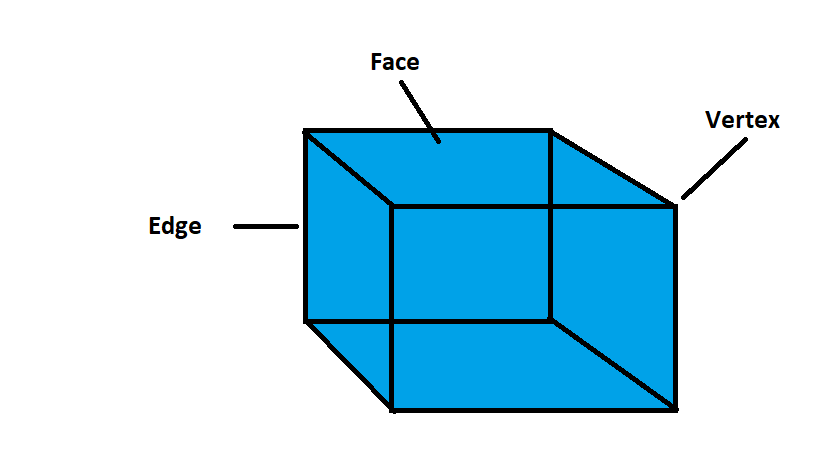Face is a flat surface that forms part of the boundary of a solid object.

A cube has six square faces.

Q.5) Explanation:

A vertex is a corner

A cube has eight vertices.

Q.6) Explanation:

An edge is a line segment joining two vertex.

When two adjacent sides of cube meet at a line segment, called an edge of the cuboid

A cube has 12 edges.

Q.7) Explanation: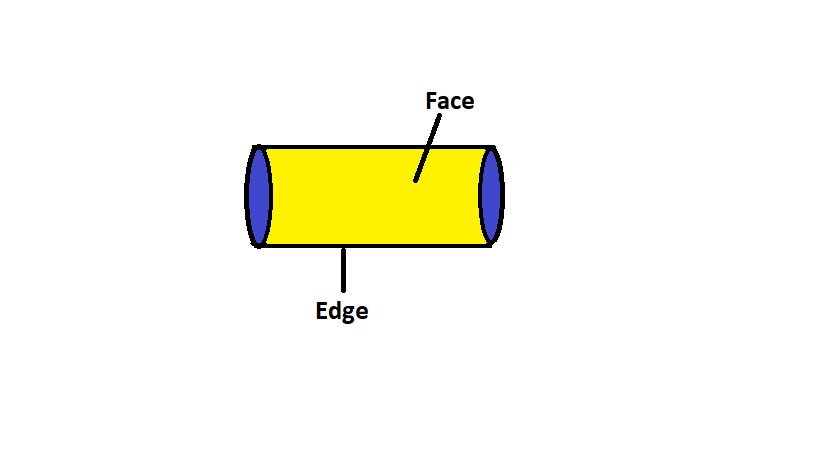Face is a flat surface that forms part of the boundary of a solid object.

A cylinder has a curved lateral surface and two circular faces at its ends.

Hence, total number of faces in cylinder is 3.

Q.8) Explanation:

A vertex is a corner

A cylinder has no corner or vertex.

Q.9) Explanation:

An edge is a line segment joining two vertex.

A cylinder has 2 circular edges.

Q.10) Explanation: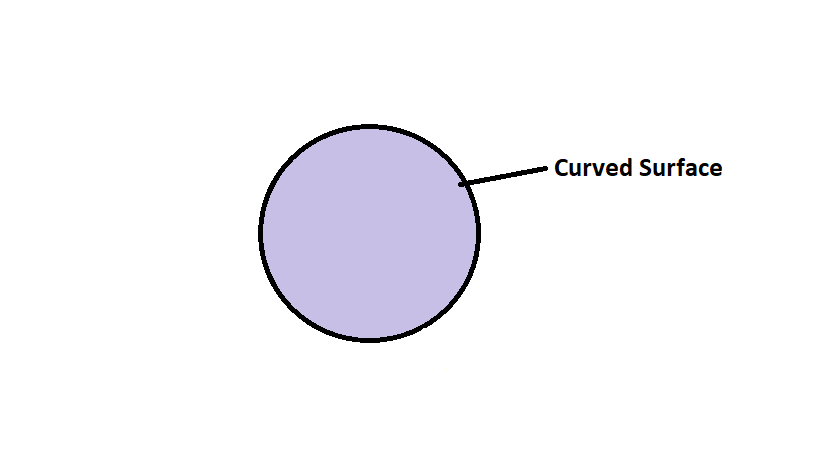Face is a flat surface that forms part of the boundary of a solid object.

A sphere has a curved surface

Q.11) Explanation:

A vertex is a corner

A sphere has no vertex.

Q.12) Explanation:

An edge is a line segment joining two vertex.

A sphere has no edges.

Q.13) Explanation: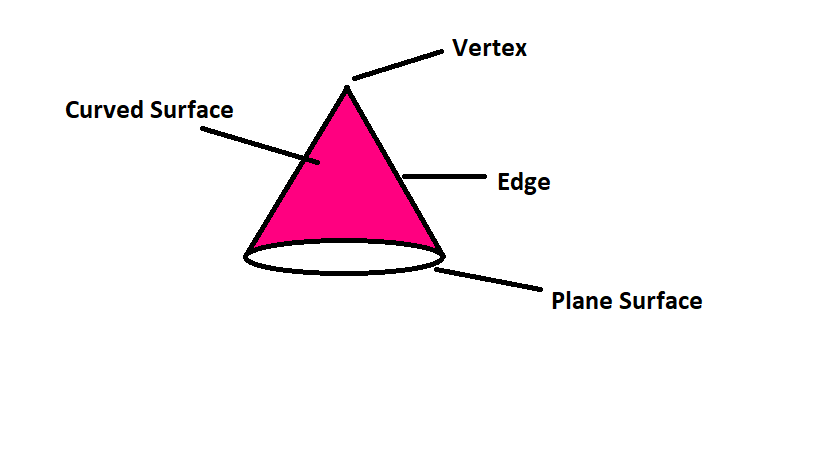Face is a flat surface that forms part of the boundary of a solid object.

A cone has one plane surface (i.e base) and one curved face

Hence, a cone has 2 faces

Q.14) Explanation:

A vertex is a corner

A cone has 1 vertex.

Q.15) Explanation:

An edge is a line segment joining two vertex.

A cone has 1 circular edge.

Q.16) Explanation: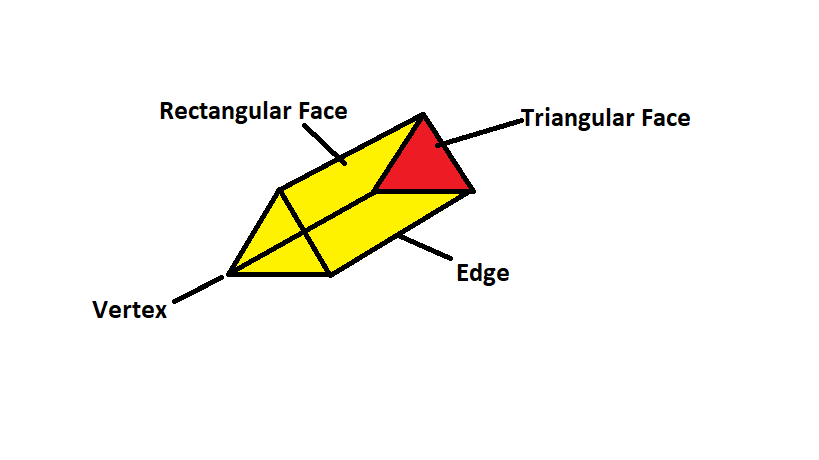Face is a flat surface that forms part of the boundary of a solid object.

A triangular prism has 3 rectangular lateral faces and 2 triangular bases.

Q.17) Explanation:

A vertex is a corner

A triangular prism has 6 vertices.

Q.18) Explanation:

An edge is a line segment joining two vertex.

A triangular prism has 9 edges.

Q.19) Explanation: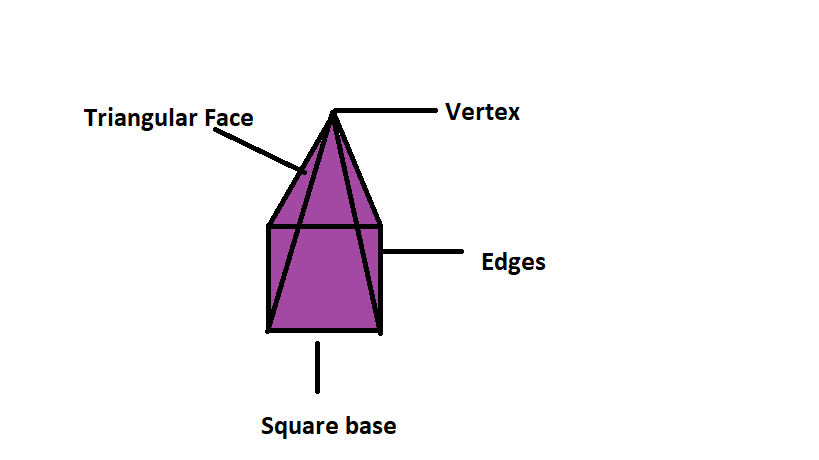Face is a flat surface that forms part of the boundary of a solid object.

A square pyramid has 4 lateral triangular faces and 1 square base.

Q.20) Explanation:

A vertex is a corner

A square pyramid has 5 vertices.

Q.21) Explanation:

An edge is a line segment joining two vertex.

A square pyramid 8 edges.

Q.22) Explanation: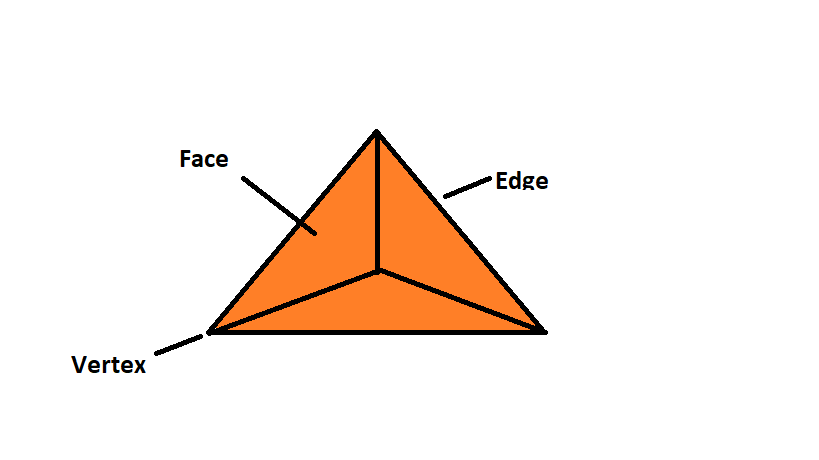Face is a flat surface that forms part of the boundary of a solid object.

A triangular pyramid has 3 triangular lateral faces, 1 triangular base

Q.23) Explanation:

A vertex is a corner

A triangular pyramid has 4 vertices.

Q.24) Explanation:

An edge is a line segment joining two vertex.

A triangular pyramid has 6 edges.